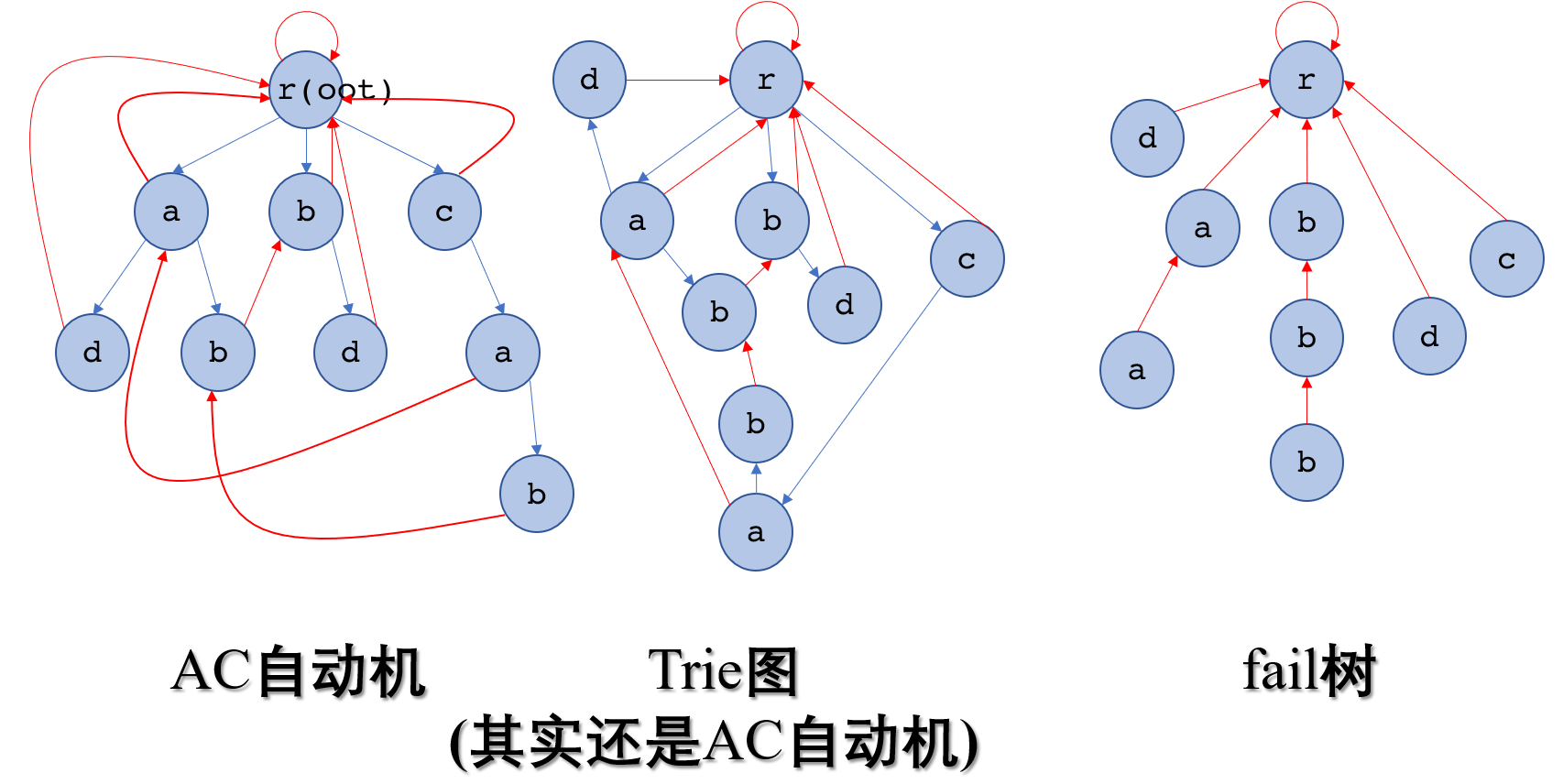# AC自动机 学习笔记【AC自动机】【字符串】【字典树】

AC 自动机是一种方便的多模式串匹配算法。基于字典树，用到了类似KMP的思维。

AC 自动机与 KMP 不同的是，AC 自动机可以同时匹配多个模式串，而复杂度不会达到太高。如果用 KMP 多次匹配字符串，复杂度就是 $O(k(n+m))$。

KMP 中的 nxt 数组是由变量 j 承接了前一个位置的 nxt，我们考虑在 AC 自动机中也让失配指针从父节点转移过来。那么如此一来，当前节点（设为’c’）的失配指针就会从当前父节点的失配指针一直沿失配指针递归，找到第一个有以’c’字符为儿子的节点，把当前节点的失配指针连接到这个节点的’c’儿子上。如此做下去，会发现有了失配指针的树变成了一个图。但是如果上面的回溯过程找到根了还没有找到怎么办？UPD:如果每个模式串答案只统计一次，那么可以在访问到一个已经被访问的节点后直接返回，因为后面连接的fail指针的答案已经被统计过了，也就没有必要统计。用到了记忆化搜索的思想。

UPD on 2019.5.6

void match()
{
int ans=0;
scanf("%s",s);
node *now=root;
for(int i=0;s[i]!='\0';++i)
{
now=now->ch[s[i]-'a'];
ans+=now->End;
now->End=0;//End置为0表示以后不再做出贡献
node *p=now;
while(p!=root)
{
p=p->fail;
ans+=p->End;//同理
}
}
printf("%d\n",ans);
}


void match()
{
int ans=0;
scanf("%s",s);
node *now=root;
for(int i=0;s[i]!='\0';++i)
{
now=now->ch[s[i]-'a'];
if(~now->End)//如果是-1就不统计答案
ans+=now->End;
now->End=-1;
node *p=now;
while(p!=root&&~p->End)
{
p=p->fail;
ans+=p->End;
p->End=-1;//置为-1
}
}
printf("%d\n",ans);
}


Code of luoguP3796：AC自动机加强版

#include<cstdio>
#include<cstring>
#include<vector>
using std::vector;
vector<int> same;//与某一个模式串相同的模式串编号
struct node
{
int End,num;//num表示相同模式串个数，End表示是否为结束位置
node *ch;
node *fail;
node()
{
memset(ch,0,sizeof(ch));
fail=NULL;
End=0;
num=0;
}
void build(char *c,int i)//构建字典树
{
if(*c=='\0')
{
End=1;
if(!num)
num=i;
same[num].push_back(i);//如果发现这里已经有单词结束了，那么一定是重复的，直接向原来的后面加编号就好了
return;
}
if(!ch[*c-'a'])
ch[*c-'a']=new node();
ch[*c-'a']->build(c+1,i);
}
}*root=new node();
char t;
node *q;//用队列完成BFS
int l=0,r=0;
void Fail()//构建fail指针
{
root->fail=root;//没有这句话貌似也可以，为了保险起见，防止越界
for(int i=0;i<26;++i)//根节点的儿子失配指针都指向自己
if(!root->ch[i])//没有这个儿子就指向失配指针的这个儿子，而失配指针是自己，为了不紊乱和方便，这个儿子就指向自己
root->ch[i]=root;
else
{
root->ch[i]->fail=root;//设置失配指针
q[++r]=root->ch[i];
}
while(l<r)
{
node *p=q[++l];
for(int i=0;i<26;++i)
if(p->ch[i])
{
p->ch[i]->fail=p->fail->ch[i];//有这个儿子就设置失配指针到自己的失配指针，自己的失配指针指向的地方一定已经完成工作了
q[++r]=p->ch[i];
}
else
p->ch[i]=p->fail->ch[i];
}
return;
}
char s;
int cnt;
void match()
{
int ans=0;
scanf("%s",s);
node *now=root;
for(int i=0;s[i]!='\0';++i)//开始匹配
{
now=now->ch[s[i]-'a'];
cnt[now->num]+=now->End;
node *p=now;
while(p!=root)//暴力跳fail
{
p=p->fail;
cnt[p->num]+=p->End;
}
}
}
int main()
{
int n;
scanf("%d",&n);
while(n)
{
root=new node();
memset(cnt,0,sizeof(cnt));
for(int i=1;i<=n;++i)
{
scanf("%s",t[i]);
root->build(t[i],i);
}
Fail();
match();
int mx=0;
for(int i=1;i<=n;++i)
{
for(vector<int>::iterator it=same[i].begin();it!=same[i].end();++it)//处理相同模式串
cnt[*it]=cnt[i];
if(mx<cnt[i])
{
cnt=1;
mx=cnt[i];
}
else if(mx==cnt[i])
++cnt;
}
printf("%d\n",mx);
for(int i=1;i<=n;++i)
if(cnt[i]==mx)
printf("%s\n",t[i]);
scanf("%d",&n);
}
return 0;
}


### 11 说点什么0 Followers

Most reacted comment
0 Comment authors
Recent comment authors
Subscribereal social signals[…] There you can find 46759 more Information to that Topic: wjyyy.top/1513.html […][…] Find More on to that Topic: wjyyy.top/1513.html […][…] Here you can find 18162 more Information to that Topic: wjyyy.top/1513.html […][…] There you will find 95282 additional Info on that Topic: wjyyy.top/1513.html […][…] Find More to that Topic: wjyyy.top/1513.html […][…] There you can find 80279 more Information on that Topic: wjyyy.top/1513.html […][…] Find More here to that Topic: wjyyy.top/1513.html […]wpDiscuz